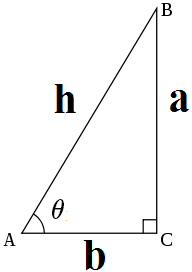# # 三角函数

## # 定义

### # 几何定义## # 勾股定理及推论

(P.S. 多提一嘴，目前没并没有可靠的历史记录了中国人证明了勾股定理，其实古代很多文明都发现了一些勾股数，但可考的给出严格逻辑证明的第一人是古希腊的毕达哥拉斯，其弟子还因此发现了无理数。即使不很可靠的《周髀算经》成书年代也比毕达哥拉斯晚 700-800 年。)

## # 常见三角函数的值域、周期与图象

### # 正弦### # 余弦### # 正切## # 通用编程实现

### # JS 实现

// 计算前 10 项展开式
function sin (x) {
const max = 10
const factorial = (n) => {
if (n < 2) return 1
return n * factorial(n - 1)
}
let sum = 0
for (let n = 0; n < max; n++) {
sum += ((-1) ** n) * (x ** (2 * n + 1)) / factorial(2 * n + 1)
}
return sum
}
console.log(sin(128 * (Math.PI/180))) // x 是弧度值，要把角度转成弧度
// 0.788010753606307


### # Python 复数实现

import math
e = math.e
pi = math.pi
def sin (x):
# j 是 Python 里的虚数单位，编程语言里习惯使用 j 而不是 i
return (e **(1j * x) - e **(-1j * x)) / 2j
print(sin(128 * pi/180))

(0.788010753606722+0j)